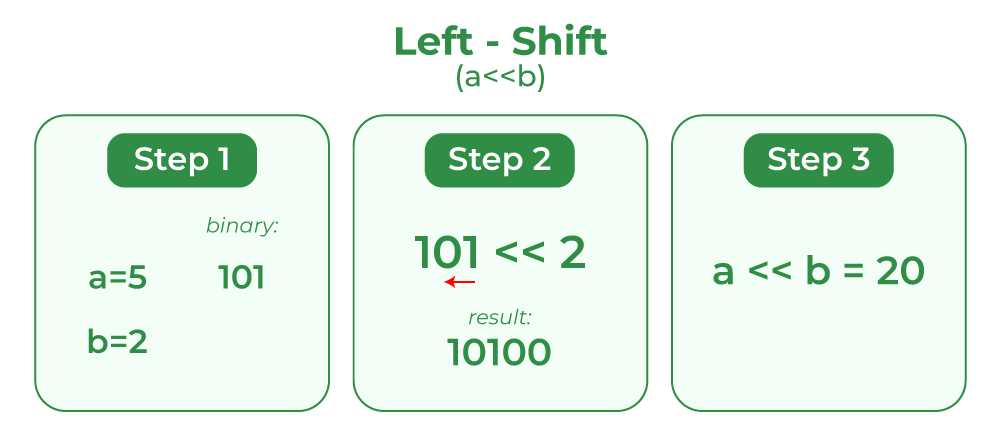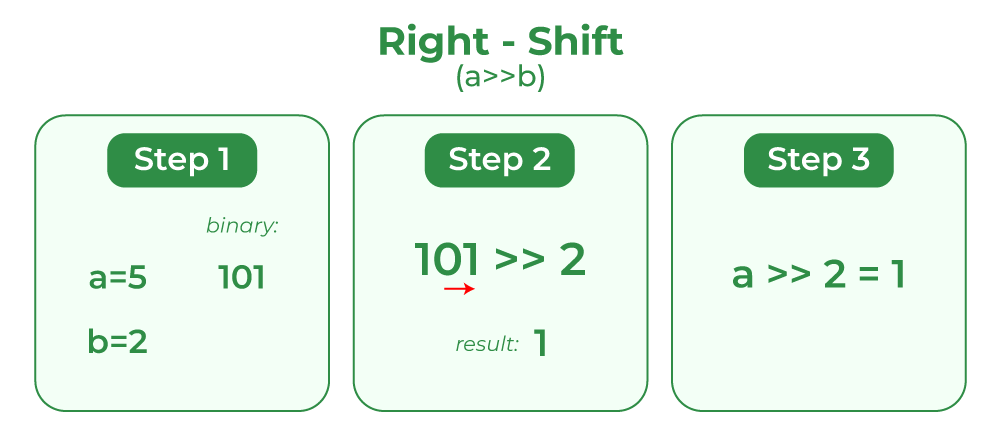Open in App
Not now

# Left Shift and Right Shift Operators in C/C++

• Difficulty Level : Easy
• Last Updated : 17 Mar, 2023

## Left Shift(<<)

It is a binary operator that takes two numbers, left shifts the bits of the first operand, and the second operand decides the number of places to shift. In other words, left-shifting an integer “a” with an integer “b” denoted as ‘(a<<b)’ is equivalent to multiplying a with 2^b (2 raised to power b).

Syntax:

`a << b;`
• a: First Operand
• b: Second Operand

Example: Let’s take a=5; which is 101 in Binary Form. Now, if “a is left-shifted by 2” i.e a=a<<2 then a will become a=a*(2^2). Thus, a=5*(2^2)=20 which can be written as 10100.## C

 `// C Program to demonstrate use` `// of left shift  operator` `#include `   `// Driver code` `int` `main()` `{` `  ``// a = 5(00000101), b = 9(00001001)` `  ``unsigned ``char` `a = 5, b = 9;` `  `  `  ``// The result is 00001010` `  ``printf``(``"a<<1 = %d\n"``, (a << 1));` `  `  `  ``// The result is 00010010` `  ``printf``(``"b<<1 = %d"``, (b << 1));` `  ``return` `0;` `}`

## C++

 `// C++ Program to demonstrate use` `// of left shift  operator` `#include ` `using` `namespace` `std;`   `// Driver code` `int` `main()` `{` `  ``// a = 5(00000101), b = 9(00001001)` `  ``unsigned ``char` `a = 5, b = 9;` `  `  `  ``// The result is 00001010` `  ``cout << ``"a<<1 = "` `<< (a << 1) << endl;` `  `  `  ``// The result is 00010010` `  ``cout << ``"b<<1 = "` `<< (b << 1) << endl;` `  ``return` `0;` `}`

Output

```a<<1 = 10
b<<1 = 18```

## Right Shift(>>)

It is a binary operator that takes two numbers, right shifts the bits of the first operand, and the second operand decides the number of places to shift. In other words, right-shifting an integer “a” with an integer “b” denoted as ‘(a>>b)‘ is equivalent to dividing a with 2^b.

Syntax:

`a >> b;`
• a: First Operand
• b: Second Operand

Example: let’s take a=5; which is 101 in Binary Form. Now, if “a is right-shifted by 2i.e a=a>>2 then a will become a=a/(2^2). Thus, a=a/(2^2)=1 which can be written as 01.## C

 `// C Program to demonstrate ` `// use of right-shift operator` `#include `   `// Driver code` `int` `main()` `{` `  ``// a = 5(00000101), b = 9(00001001)` `  ``unsigned ``char` `a = 5, b = 9;`   `  ``// The result is 00000010` `  ``printf``(``"a<<1 = %d\n"``, (a << 1));`   `  ``// The result is 00000100` `  ``printf``(``"b<<1 = %d"``, (b << 1));` `  `  `  ``return` `0;` `}`

## C++

 `// C++ Program to demonstrate ` `// use of right-shift operator` `#include ` `using` `namespace` `std;`   `// Driver code` `int` `main()` `{` `  ``// a = 5(00000101), b = 9(00001001)` `  ``unsigned ``char` `a = 5, b = 9;`   `  ``// The result is 00000010` `  ``cout << ``"a>>1 = "` `<< (a >> 1) << endl;`   `  ``// The result is 00000100` `  ``cout << ``"b>>1 = "` `<< (b >> 1) << endl;` `  `  `  ``return` `0;` `}`

Output

```a>>1 = 2
b>>1 = 4```

## Important Points

1. The left-shift and right-shift operators should not be used for negative numbers. The result of is undefined behavior if any of the operands is a negative number. For example results of both 1 >> -1 and 1 << -1 is undefined.

## C

 `// C program to show behaviour of shift operators for` `// negative values` `#include `   `int` `main()` `{` `      ``// left shift for negative value` `    ``printf``(``"2 << -5 = %d\n"``, (2 << -5));` `      `  `      ``//    right shift for negative value` `      ``printf``(``"2 >> -5 = %d"``, (2 >> -5));` `  `  `      ``return` `0;` `}`

## C++

 `// C++ program to show behaviour of shift operators for` `// negative values` `#include `   `using` `namespace` `std;`   `int` `main()` `{` `    ``// left shift for negative value` `    ``cout << ``"2 << -5 = "` `<< (2 << -5) << endl;`   `    ``//    right shift for negative value` `    ``cout << ``"2 >> -5 = "` `<< (2 >> -5) << endl;`   `    ``return` `0;` `}`

Output

```2 << -5 = 0
2 >> -5 = 64```

2. If the number is shifted more than the size of the integer, the behavior is undefined. For example, 1 << 33 is undefined if integers are stored using 32 bits. For bit shift of larger values 1ULL<<62  ULL is used for Unsigned Long Long which is defined using 64 bits that can store large values.

## C

 `//    c program to demonstrate the behaviour of bitwise` `//shift operators for large values` `#include `   `int` `main()` `{` `    ``int` `N = 3;`   `    ``// left shift of 65 digits` `    ``printf``(``"3 << 65 = %d"``, (3 << 65));`   `    ``return` `0;` `}`

## C++

 `//    c++ program to demonstrate the behaviour of bitwise` `//shift operators for large values` `#include `   `using` `namespace` `std;`   `int` `main()` `{` `      ``int` `N = 3;` `  `  `      ``// left shift by 65 digits` `      ``cout << ``"3 << 65"` `<< (3 << 65) << endl;` `  `  `    ``return` `0;` `}`

Output

`3 << 65 = 0`

3. The left-shift by 1 and right-shift by 1 are equivalent to the product of the first term and 2 to the power given element(1<<3 = 1*pow(2,3)) and division of the first term and second term raised to power 2 (1>>3 = 1/pow(2,3)) respectively.

## C++

 `// C++ program to get the shifted values using pow()` `#include ` `#include `   `using` `namespace` `std;`   `int` `main()` `{` `      ``cout << ``"2^5 using pow() function"` `<< ``pow``(2, 5) << endl;` `  `  `      ``cout << ``"2^5 using leftshift"` `<< (1 << 5) << endl;` `  `  `      ``return` `0;` `}`

## C++

 `// C program for the above approach `   `#include ` `#include `   `int` `main()` `{` `    ``printf``(``"2^5 using pow() function: %.0f\n"``, ``pow``(2, 5));` `    ``printf``(``"2^5 using left shift: %d\n"``, (1 << 5));` `    ``return` `0;` `}`   `// This code is contributed Prince Kumar`

Output

```2^5 using pow() function32
2^5 using leftshift32
```

Must Read: Bitwise Operators in C/C++

My Personal Notes arrow_drop_up
Related Articles